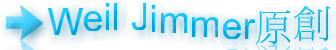#### Category：Experience

Found 42 records. Now is Page 7 / 9.

## JavaScript XML Dom 標籤名稱有冒號的情況 getElementsByTagNameNo Comments

JavaScript 是

``xmlDoc.getElementsByTagName("encoded").childNodes.nodeValue;``

This entry was posted in By Weil Jimmer.

This entry was posted in By Weil Jimmer.

====================

So, 正常的時空，正常的邏輯，姑且叫這個正常的世界為「世界A」。

So, 第二集又再次改變歷史，那我們再次稱這個世界為 世界B 吧。

=============

(很奇特吧，這樣怎麼能說 反抗軍打敗了天網。邏輯不通，但是這不是世界A，也不是世界B，而是 一個新的世界C，所以發生這種事情應該也很正常。就是，康納沒有打敗天網。)

==>既然天網做出T5000，可以感染人類，那天網怎麼可以算是被打敗。科技太先進，看這部片，只會讓我思考，T3000到底是什麼東西！太強大了，有人說T1000感覺比康納厲害，我反倒不覺得，我認為最強的還是T3000、T5000是超級BOSS，感染了康納。

7/10分吧。This entry was posted in By Weil Jimmer.

## int 和 Integer 的比較 Java 程式設計No Comments

``````int test_int_1 = 1;
int test_int_2 = 1;
System.out.println(test_int_1==test_int_2);//true
int test_int_3 = new Integer(1);
int test_int_4 = new Integer(1);
System.out.println(test_int_3==test_int_4);//true
int test_int_5 = new Integer("1");
int test_int_6 = new Integer("1");
System.out.println(test_int_5==test_int_6);//true
int test_int_7 = Integer.valueOf(1);
int test_int_8 = Integer.valueOf(1);
System.out.println(test_int_7==test_int_8);//true
int test_int_9 = Integer.valueOf("1");
int test_int_10 = Integer.valueOf("1");
System.out.println(test_int_9==test_int_10);//true
Integer test_integer_1 = 1;
Integer test_integer_2 = 1;
System.out.println(test_integer_1==test_integer_2);//true
System.out.println(test_integer_1.equals(test_integer_2));//true
Integer test_integer_3 = new Integer("1");
Integer test_integer_4 = new Integer("1");
System.out.println(test_integer_3==test_integer_4);//false
System.out.println(test_integer_3.equals(test_integer_4));//true
Integer test_integer_5 = Integer.valueOf("1");
Integer test_integer_6 = Integer.valueOf("1");
System.out.println(test_integer_5==test_integer_6);//true
System.out.println(test_integer_5.equals(test_integer_6));//true``````

``new Integer("1")==new Integer("1");//false``

``````test_integer_6 = Integer.valueOf("1");
Integer test_integer_7 = Integer.valueOf(1);
System.out.println(test_integer_6==test_integer_7);//true
System.out.println(test_integer_6.equals(test_integer_7));//true

System.out.println(test_integer_1==test_integer_3);//false
System.out.println(test_integer_1.equals(test_integer_3));//true
System.out.println(test_integer_5==test_integer_3);//false
System.out.println(test_integer_5.equals(test_integer_3));//true
System.out.println(test_integer_7==test_integer_3);//false
System.out.println(test_integer_7.equals(test_integer_3));//true``````

``````Integer test_special_1 = Integer.valueOf(127);//等於Integer test_special_1 = 127;
Integer test_special_2 = Integer.valueOf(127);
System.out.println(test_special_1==test_special_2);//true
System.out.println(test_special_1.equals(test_special_2));//true
Integer test_special_3 = Integer.valueOf(128);//等於Integer test_special_1 = 128;
Integer test_special_4 = Integer.valueOf(128);
System.out.println(test_special_3==test_special_4);//false
System.out.println(test_special_3.equals(test_special_4));//true``````

This entry was posted in By Weil Jimmer.

``List<String> list = new LinkedList<String>(Arrays.asList(split));``

This entry was posted in By Weil Jimmer.

### Visitor Countnonenonenone

### Note

Support Net Neutrality.

Celebration for General Data Protection Regulation.

### Counter

2835The strong do what they can and the weak suffer what they must.

Privacy is your right and ability to be yourself and express yourself without the fear that someone is looking over your shoulder and that you might be punished for being yourself, whatever that may be.

It is quality rather than quantity that matters.

I WANT Internet Freedom.

Reality made most of people lost their childishness.

Justice,Freedom,Knowledge.

Without music life would be a mistake.

### Support/Donate

 This site also need a little money to maintain operations, not entirely without any cost in the Internet. Your donations will be the best support and power of the site. Method Bitcoin AddressLinode

### Categories

Android (7)

Announcement (4)

Arduino (2)

Bash (2)

C (3)

C# (5)

C++ (1)

Experience (42)

Flash (2)

Free (13)

Functions (36)

Games (13)

General (50)

HTML (7)

Java (13)

JS (7)

Mood (24)

Note (28)

Office (1)

PHP (9)

Privacy (4)

Product (12)

Python (4)

Software (11)

The Internet (16)

Tools (14)

VB.NET (8)

WebHosting (7)

Wi-Fi (5)

XML (4)